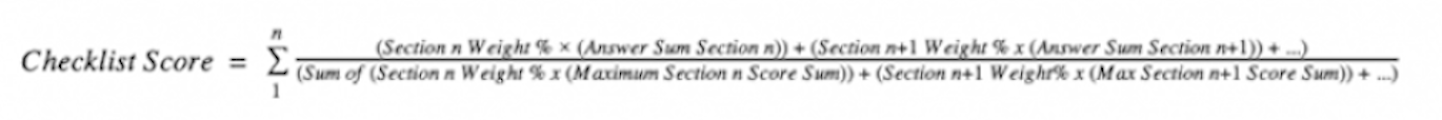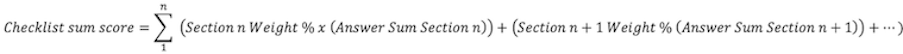Checklist scoring is assigned at the answer level. You can add point values to individual answer choices to add another layer of analysis to your checklist designs. When you assign point values to answer choices, Vault provides a total score when the user completes the checklist. keeps track of the running score as users answer questions and provide a total score when they complete the checklist. Vault provides the checklist score as a percentage and a sum score.

You can assign weighting at the section level and, for aggregate checklists, at the sub-checklist level. You can assign weighted percentages to different sections of your checklist. Vault runs validation to ensure weighted section totals equal 100% before you can make the checklists available for users. For aggregate checklists, you can assign weighted percentages to different sub checklists, and Vault runs validation to ensure the weighted sub-checklist totals equal 100%. You can still assign a weighted percentage to a sub-checklist even if the sub-checklist itself is not weighted but has at least one multiple choice question.

### How to Configure Question Scoring

You can configure question answer scores for each answer option of a multiple choice question. Enter any positive or negative numerical value or zero (0) as the Score. Scoring is not available for text, date, or number questions.

### How to Configure Weighting for Sections and Sub Checklists

Set weighted percentages for question sections from the Sections area of the Checklist Design object details page. Remember that all sections must total 100% before you can make the checklist active.

For Checklist Designs with Aggregate Checklists set to Yes, set weighted percentages for sub checklists from the Sub-Checklist Designs area of the Checklist Design object details page. Remember that all sub checklists must total 100% before you can make the checklist active. Sub checklists with a weight must have at least one multiple choice question.

## Understanding Score Calculations

The Section score and Checklist score are calculated when the Checklist moves to the Completed lifecycle state, and persisted in the Target Object Section and Target Object Checklist runtime objects.

For multiple choice questions that are the checkbox (multi-select) checklist answer type, the score for the question is the sum of the scores for each selected option.

The Target Object Section and Target Object Checklist contains two fields that calculate the total score of the Checklist and Section scores:

• Score %: A percentage value of the total score. The percentage is calculated using the sum of the scores for each selected multiple choice answer and the sum of the maximum possible scores for each question. If the answer score has negative values assigned and the sum of the answer scores for each section is less than zero (0), Vault sets the answer sum to zero (0) for that section. Vault also checks that the maximum score sum for a section is greater than or equal to zero (0).

• Sum Score: The total score represented as a whole number or a decimal number for weighted scores. The sum score is calculated using only the total sum of the scores for each selected multiple choice answer. Vault displays a negative value (100, -50, -20) in the Sum Score field if the sum of the answer scores for each section is less than zero.

### Scoring Calculations

Vault uses certain formulas to calculate the sum score and score percentage. The formulas used are defined as follows:

• `(answer sum)` is the sum of the scores for each selected multiple choice answer.
• `(maximum score sum)` is the sum of the maximum possible scores for each question.
• `(section n weight %)` is the specified weight percentage for a section.
• `(answer sum section n)` is the sum of the scores for each answer in that section.
• `(maximum section n score sum)` is the sum of the maximum possible scores for each answer in that section.

### Non-Weighted and Weighted Score % Calculation

If the Checklist Design is not weighted, the Section and Checklist score percentage are each calculated as follows: `((answer sum) / (maximum score sum))`

If the Checklist Design is weighted, the Section score percentage is calculated as follows: `((answer sum) / (maximum score sum))`

And the Checklist score percentage is calculated as follows:### Non-Weighted and Weighted Sum Score Calculation

If the Checklist Design is not weighted, the sum score of the Section and Checklist are each calculated as follows: `(answer sum)`

If the Checklist Design is weighted, the sum score of the Section is calculated as follows:### Aggregate Checkclist Scoring

For aggregate checklists, percentage and sum scores are calculated in the same way as non-aggregate checklists, with sub-checklist scores calculated in the same way as Sections; however, if the sub-checklist is weighted then weighted is respected in the sub-checklist calculation.

### Scoring Example

The checklist below contains two sections with two multiple choice questions each. The bolded answers represent the selected multiple choice answers. The questions in this example are not checkbox multiple choice questions.

Section 1

• Question 1
• Question 2

Section 2

• Question 1
• Question 2